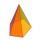# Tetrahedral pyramid

Calculate the volume and surface area of a regular tetrahedral pyramid, its height is \$b cm and the length of the edges of the base is 6 cm.

Result

S =  196.1 cm2
V =  156 cm3

#### Solution:

$a = 6 \ cm \ \\ h = 13 \ cm \ \\ s = \sqrt{ h^2+(a/2)^2 } = \sqrt{ 13^2+(6/2)^2 } = \sqrt{ 178 } \ cm \doteq 13.3417 \ cm \ \\ S_{ 1 } = a \cdot \ s/2 = 6 \cdot \ 13.3417/2 = 3 \ \sqrt{ 178 } \ cm^2 \doteq 40.025 \ cm^2 \ \\ \ \\ S = a^2 + 4 \cdot \ S_{ 1 } = 6^2 + 4 \cdot \ 40.025 \doteq 196.1 = 196.1 \ cm^2$
$V = \dfrac{ 1 }{ 3 } \cdot \ a^2 \cdot \ h = \dfrac{ 1 }{ 3 } \cdot \ 6^2 \cdot \ 13 = 156 = 156 \ cm^3$

Leave us a comment of this math problem and its solution (i.e. if it is still somewhat unclear...):Be the first to comment!#### Following knowledge from mathematics are needed to solve this word math problem:

Pythagorean theorem is the base for the right triangle calculator. Do you want to convert area units? Do you know the volume and unit volume, and want to convert volume units? See also our trigonometric triangle calculator.

## Next similar math problems:

1. Tetrahedral pyramidCalculate the volume and surface of the regular tetrahedral pyramid if content area of the base is 20 cm2 and deviation angle of the side edges from the plane of the base is 60 degrees.
2. Tetrahedral pyramidWhat is the surface of a regular tetrahedral (four-sided) pyramid if the base edge a=16 and height v=16?
3. Pyramid roof2/4 of area of ​​the roof shaped regular tetrahedral pyramid with base edge 10 m and height of 4 m is already covered with roofing. How many square meters still needs to be covered?
4. 4s pyramidRegular tetrahedral pyramid has a base edge a=17 and collaterally edge length b=32. What is its height?
5. Triangular pyramidCalculate the volume and surface area of a regular triangular pyramid whose height is equal to the length of the base edges 10 cm.
6. Hexagonal pyramidCalculate the volume and the surface of a regular hexagonal pyramid with a base edge length of 3 cm and a height of 5 cm.
7. 4side pyramidCalculate the volume and surface of 4 sides regular pyramid whose base edge is 4 cm long. The angle from the plane of the sidewall and base plane is 60 degrees.
8. Pentagonal pyramidCalculate the volume of a regular 5-side (pentaprism) pyramid ABCDEV; if |AB| = 7.7 cm and a plane ABV, ABC has angle 37 degrees.
9. Square pyramidCalculate the volume of the pyramid with the side 5cm long and with a square base, side-base has angle of 60 degrees.How many square meters is needed to cover the tower the shape of regular quadrangular pyramid base edge 10 meters, if the deviation lateral edges from the base plane is 68 °? Calculate coverage waste 10%.
11. Pyramid - angleCalculate the surface of regular quadrangular pyramid whose base edge measured 6 cm and the deviation from the plane of the side wall plane of the base is 50 degrees.
12. House roofThe roof of the house has the shape of a regular quadrangular pyramid with a base edge 17 m. How many m2 is needed to cover roof if roof pitch is 57° and we calculate 11% of waste, connections and overlapping of area roof?
13. PyramidPyramid has a base a = 5cm and height in v = 8 cm. a) calculate angle between plane ABV and base plane b) calculate angle between opposite side edges.
14. Triangular pyramidIt is given perpendicular regular triangular pyramid: base side a = 5 cm, height v = 8 cm, volume V = 28.8 cm3. What is it content (surface area)?
15. Secret treasureScouts have a tent in the shape of a regular quadrilateral pyramid with a side of the base 4 m and a height of 3 m. Determine the radius r (and height h) of the container so that they can hide the largest possible treasure.
16. FlowerbedFlowerbed has the shape of a truncated pyramid, the bottom edge of the base a = 10 m, the upper base b = 9 m. Deviation angle between edge and the base is alpha = 45°. What volume is needed to make this flowerbed? How many plants can be planted if 1 m2 =.
17. TowerThe top of the tower is a regular hexagonal pyramid with base edge 8 meters long and a height 5 meters. How many m2 of the sheet is required to cover the top of the tower if we count 8% of the sheet waste?